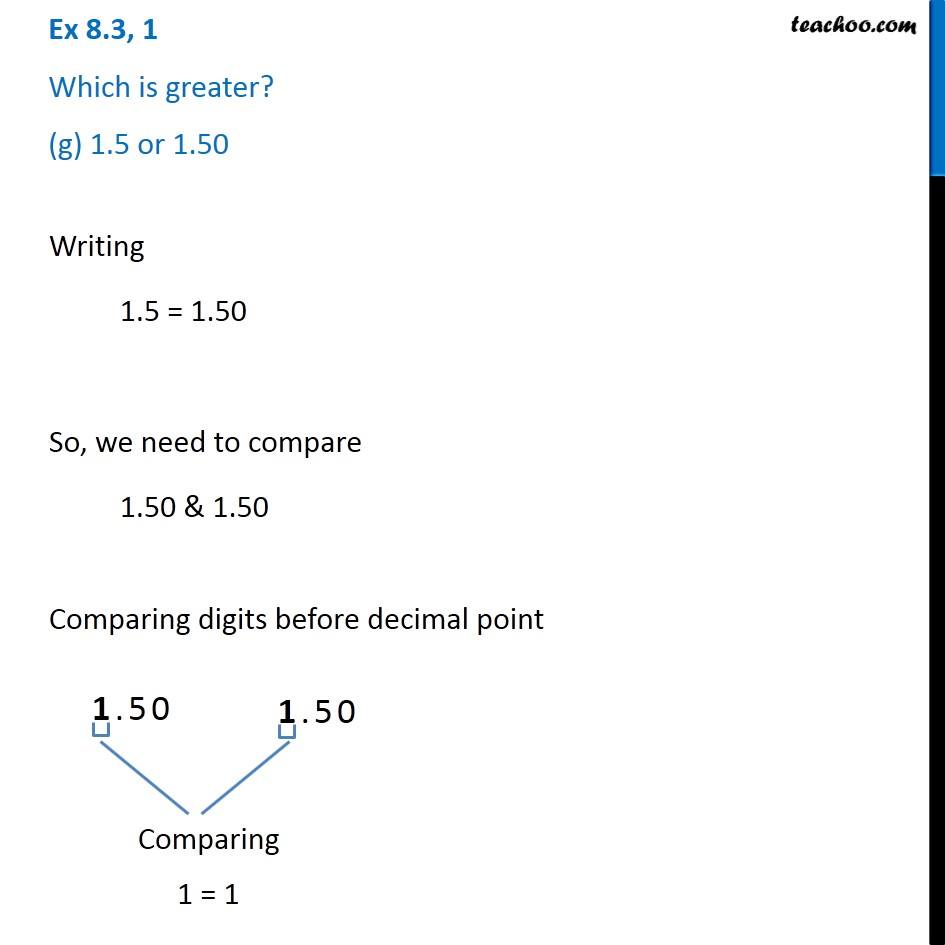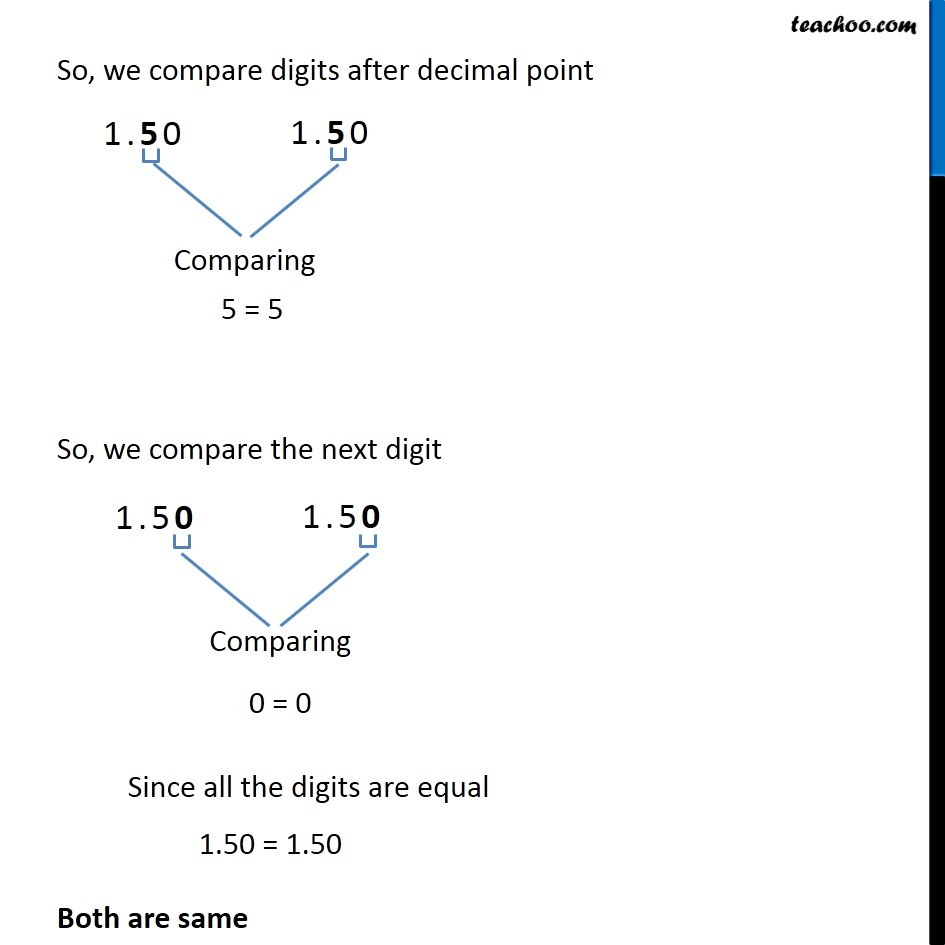1. Chapter 8 Class 6 Decimals
2. Concept wise
3. Comparing decimals

Transcript

Ex 8.3, 1 Which is greater? (g) 1.5 or 1.50 Writing 1.5 = 1.50 So, we need to compare 1.50 & 1.50 Comparing digits before decimal point 1 = 1 So, we compare digits after decimal point 5 = 5 So, we compare the next digit 0 = 0 Since all the digits are equal 1.50 = 1.50 Both are same

Comparing decimals